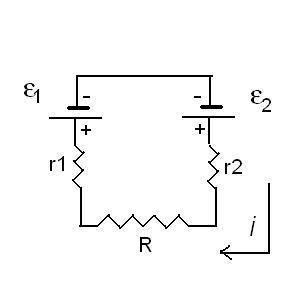# Calculating the value of R in a circuit

## Homework Statement

I have drawn the circuit.
I want to calculate the value of R, knowing that

i = 50mA
ε1 = 2V
ε2 = 3V
r1 = r2 = 3 ohm

## The Attempt at a Solution

This is a closed circuit.
I imposed the current verse as clockwise.

I go through the circuit as the verse of the current from a point "a" to the same point "a".
The difference of potential is always zero.

## -ε2 -ir_2 -iR -ir_1 +ε1 = 0 ##

I find the R.
But it is not the correct answer.
What am I doing wrong?

#### Attachments

•01.jpg
8.3 KB · Views: 339

ehild
Homework Helper
I go through the circuit as the verse of the current from a point "a" to the same point "a".
The difference of potential is always zero.

## -ε2 -ir_2 -iR -ir_1 +ε1 = 0 ##

I find the R.
But it is not the correct answer.
What am I doing wrong?
The signs of the emf of the batteries.

I don-t understand why.
ε2 has different verse than the current.

ehild
Homework Helper
Your picture is unclear. Which are the positive and negative terminals of the batteries? What direction did you assume for the current? clockwise or anti-clocwise? Note that the battery with the greater emf determines the verse of the current. The current flows out from the positive terminal of that battery and flows in at the negative one.

For the current I assumed the verse as clockwise, as I said.
The problem is that I have just this drawing. I can't know anymore else about it.

CWatters
Homework Helper
Gold Member
I think there are three possible problems:

1) You made an error with the arithmetic. Check.

2) The book answer is wrong. It happens.

3) There is an error with the polarity of one of the batteries.

Try reversing the polarity of one of the batteries. It doesn't matter which one.

Last edited:
ehild
Homework Helper
Usually, the long line represents the positive terminal of a battery. If the current flows clockwise, following the current, the potential increases by 3 V across battery 2 and decreases by 2 V through battery 1:
##-ε2 -ir_2 -iR -ir_1 +ε1 = 0##. If your teacher uses the other sign convention, the current flows anti-clockwise.
What did you get for R?If I consider the current as anticlockwise I get the following equation
## -\epsilon1 - ir1 - iR - ir2 +\epsilon2 = 0 ##
And the result is ok, R = 14 ohm.

The drawing was unclear, so I assume that the positive terminals of the batteries were above (the opposite of the circuit that ehild draw above).